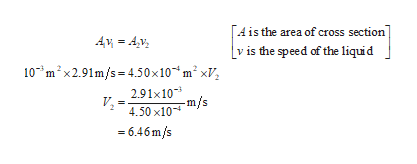# A liquid with a density of 1.65 g/cm3 flows from left to right in the pipe, from larger to smaller cross section. The left side's cross-sectional area is 10.0 cm2, and while in this side, the speed of the liquid is 2.62 m/s, and the pressure is 1.20 ✕ 105 Pa. The right side's cross sectional-area is 3.00 cm2. What is the velocity and and pressure?

Question
23 views

A liquid with a density of 1.65 g/cm3 flows from left to right in the pipe, from larger to smaller cross section. The left side's cross-sectional area is 10.0 cm2, and while in this side, the speed of the liquid is 2.62 m/s, and the pressure is 1.20 ✕ 105 Pa. The right side's cross sectional-area is 3.00 cm2. What is the velocity and and pressure?

check_circle

Step 1

The density of the liquid is 1650kg/m3. Let the area of cross section of the left end be 10.0cm2, speed of the liquid is 2.91m/s, and pressure be 1.20Pa. The area of cross section at the right end be 4.50cm2.

Step 2

(a)Write the equation ...help_outlineImage Transcriptionclose[A is the area of cross section] v is the speed of the liquid Av = 4,v, 10*m?x2.91m/s= 4.50x10*m² xV, 2.91x10- V, -m/s 4.50 x10 = 6.46m/s fullscreen

### Want to see the full answer?

See Solution

#### Want to see this answer and more?

Solutions are written by subject experts who are available 24/7. Questions are typically answered within 1 hour.*

See Solution
*Response times may vary by subject and question.
Tagged in

### Physics